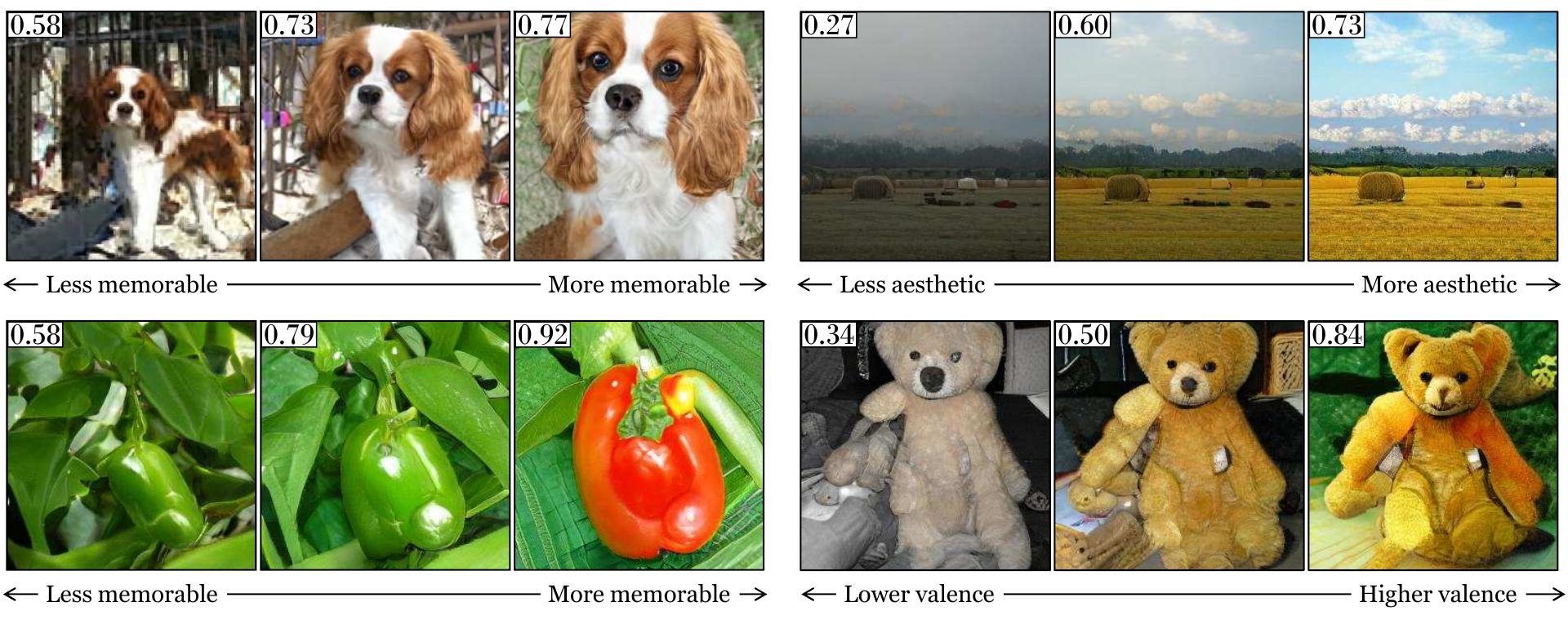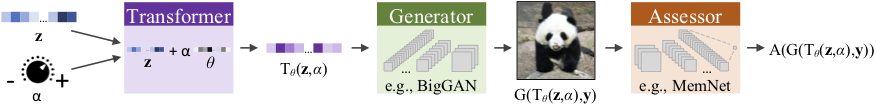# GANalyze: Toward Visual Definitions of Cognitive Image Properties

Lore Goetschalckx*12 Alex Andonian**1 Aude Oliva1 Phillip Isola1
1MIT Computer Science and Artificial Intelligence Laboratory,
2KU Leuven (Belgium)We introduce a framework that uses Generative Adversarial Networks (GANs) to study cognitive properties like memorability, aesthetics, and emotional valence. These attributes are of interest because we do not have a concrete visual definition of what they entail. What does it look like for a dog to be more or less memorable? GANs allow us to generate a manifold of natural-looking images with fine-grained differences in their visual attributes. By navigating this manifold in directions that increase memorability, we can visualize what it looks like for a particular generated image to become more or less memorable. The resulting "visual definitions" surface image properties (like "object size") that may underlie memorability. Through behavioral experiments, we verify that our method indeed discovers image manipulations that causally affect human memory performance. We further demonstrate that the same framework can be used to analyze image aesthetics and emotional valence.

## The GANalyze framework

Our framework consists of the following interacting components:

• Generator $$G$$: Given a latent noise vector $$z$$ and class label $$y$$, the generator produces a photorealistic image $$G(z, y)$$.
• Assessor $$A$$: Assigns a numerical value to an image indicating the magnitude of an cognitive property of interest.
• Transformer $$T$$: A function that moves the input $$z$$ along a certain direction $$\theta$$ in the latent space of $$G$$.

Our model learns how to transform a $$z$$ vector such that when fed to a Generator,the resulting image's property of interest changes. The transformation is achieved by the Transformer, which moves the $$z$$ vector along a learned direction, $$\theta$$, in the Generator's latent space. The property of interest (e.g., memorability) is predicted by an Assessor module (e.g., MemNet). Finally, $$\alpha$$ acts as knob to set the degree of change one wants to achieve in the Assessor value (e.g., MemNet score). It tells the Transformer how far exactly to move along $$\theta$$. The process is outlined in the schematic below.Please refer to the paper for additional details.